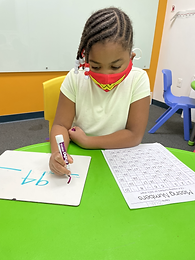## Ms. Caitlyn

### Target 1​

###### Lesson Type:

Review

Number Operation

:

Number System

Complete a number sequence from any given number zero to 100.

###### 1:

Complete a number sequence in both ascending and descending order.

###### 2:

Start a number sequence from any given number.

Kindergarden

###### Vocabulary:

Ascending, Descending, Number Order

Activities:

Students filled in empty spaces in hundreds charts that were both in ascending and descending order.### Home Exploration

###### Guiding Questions:## Absent Students:

### Target 2

:

###### 1:

Identify the correct number before or after a given number without having to review the entire count sequence.

###### 2:

Given 2 numbers, determine all the numbers that come in-between.

Kindergarden

###### Vocabulary:

Before, After, In Between

Activities:

Students reviewed the vocabulary before, after, and in-between.

Students filled in the blanks of sequences of numbers to determine what comes before, after, and in-between.### Home Exploration

###### Guiding Questions:### Target 3

:

###### 1:

Recognize the arithmetic symbols in a subtraction equation: minus (-) and equals (=).

###### 2:

Understand that subtraction means to take a quantity away from another quantity.

###### 3:

Understand that subtracting numbers means making a smaller number.

###### 4:

Understand that subtraction equals can be written both vertically and horizontally.

###### 5:

Understand that the same process is utilized to add three or more whole numbers that is used to add only two numbers.

1st

###### Vocabulary:

Activities:

Students completed a subtraction color-by-number.

Students used dots and base ten blocks to solve subtraction problems.### Home Exploration